# RBI Assistant Pre 2022 Quantitative Aptitude Quiz – 7

## RBI Assistant 2022 Quantitative Aptitude Quiz

Quantitative Aptitude measures a candidate’s numerical ability and problem-solving abilities. It is the most important section of almost all competitive exams. Candidates are often stymied by the complexity of Quantitative Aptitude Questions but if they practice more and more questions, it will become quite simple. So, here we are providing you with the RBI Assistant 2022 Quantitative Aptitude Quiz to enhance your preparation for your upcoming examination. This RBI Assistant 2022 Quantitative Aptitude Quiz is based on the most recent and the latest exam pattern. A detailed explanation for each question will be given in this RBI Assistant 2022 Quantitative Aptitude Quiz. This RBI Assistant 2022 Quantitative Aptitude Quiz is entirely free of charge. This RBI Assistant 2022 Quantitative Aptitude Quiz will assist aspirants in achieving a good score in their upcoming examinations.

1. Simple interest on a certain sum at the rate of 50/3% per annum for three years is Rs. 1250. Find the sum.
(a) Rs. 3,000
(b) Rs. 2,500
(c) Rs. 2,400
(d) Rs. 4,000
(e) Rs. 5,0002. A fraction becomes 5/3 when 20% of numerator is added to its numerator and 30% of denominator is subtracted from its denominator. Find the fraction.
(a) 35/36
(b) 36/25
(c) 33/35
(d) 27/35
(e) 35/333. The population a city after three years will be 21,600. If rate of increase of population per year be 20% then find the present population of the city.
(a) 12,500
(b) 16,500
(c) 14,500
(d) 10,500
(e) 11,600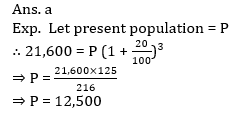4. In a school there 60% boys and rest are girls. If number of girls is 360 then find the total number of boys in the school.
(a) 540
(b) 600
(c) 900
(d) 640
(e) 480
Ans. a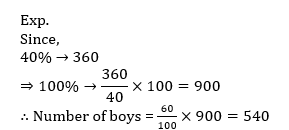5. The ratio of ages of Ravi and Shusma, three years before was 5 : 3. 2 years hence, ratio of their ages will be 4 : 3 . Find the present age of Ravi.
(a) 12 yrs 3 months
(b) 11 yrs 4 months
(c) 9 yrs 5 months
(d) 13 yrs
(e) 15 yrsDirections (6-10): Study the pie-chart given below & answer the questions.

Pie-chart given below shows the degree distribution of expenditure on various items for a hotel.6. If expenditure of Hotel on Salt is Rs 24,000 then expenditure on Sugar and rice together is how much more than expenditure on others?
(a) Rs 1200
(b) Rs 1000
(c) Rs 1600
(d) Rs 1250
(e) Rs 1450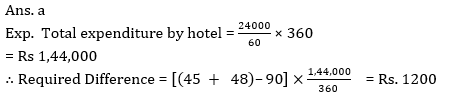7. Total expenditure on Rice and Wheat together is what percent more/less than total expenditure on Salt & oil together?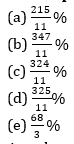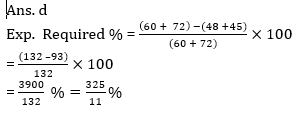8. What is the ratio of average expenditure on Salt & oil to average expenditure on Wheat & others?
(a) 43 :45
(b) 43 : 44
(c) 44 : 45
(d) None of these
(e) 3 : 59. If the total expenditure on all item is given as Rs 3,60,000 and total sugar used by hotel is 300 kg. Then find price of sugar per kg?
(a) Rs 450
(b) None of these
(c) Rs 250
(d) Rs 200
(e) Rs 150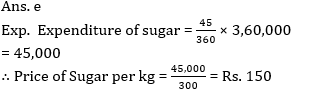10. If the total expenditure is 200% more than the total saving of the hotel and total income of hotel is Rs 5,60,000. Then find the average expenditure of hotel on salt and sugar?
(a) Rs 51,240
(b) Rs 61,250
(c) Rs 1,22,500
(d) Rs 71,250
(e) None of these#### Attempt Quantitative Aptitude Topic Wise Online Test Series

RBI Assistant Prelims Online Test Series 2022

Recommended PDF’s for:

#### Most important PDF’s for Bank, SSC, Railway and Other Government Exam : Download PDF Now

AATMA-NIRBHAR Series- Static GK/Awareness Practice Ebook PDF Get PDF here
The Banking Awareness 500 MCQs E-book| Bilingual (Hindi + English) Get PDF here
AATMA-NIRBHAR Series- Banking Awareness Practice Ebook PDF Get PDF here
Computer Awareness Capsule 2.O Get PDF here
AATMA-NIRBHAR Series Quantitative Aptitude Topic-Wise PDF Get PDF here
AATMA-NIRBHAR Series Reasoning Topic-Wise PDF Get PDF Here
Memory Based Puzzle E-book | 2016-19 Exams Covered Get PDF here
Caselet Data Interpretation 200 Questions Get PDF here
Puzzle & Seating Arrangement E-Book for BANK PO MAINS (Vol-1) Get PDF here
ARITHMETIC DATA INTERPRETATION 2.O E-book Get PDF here
3